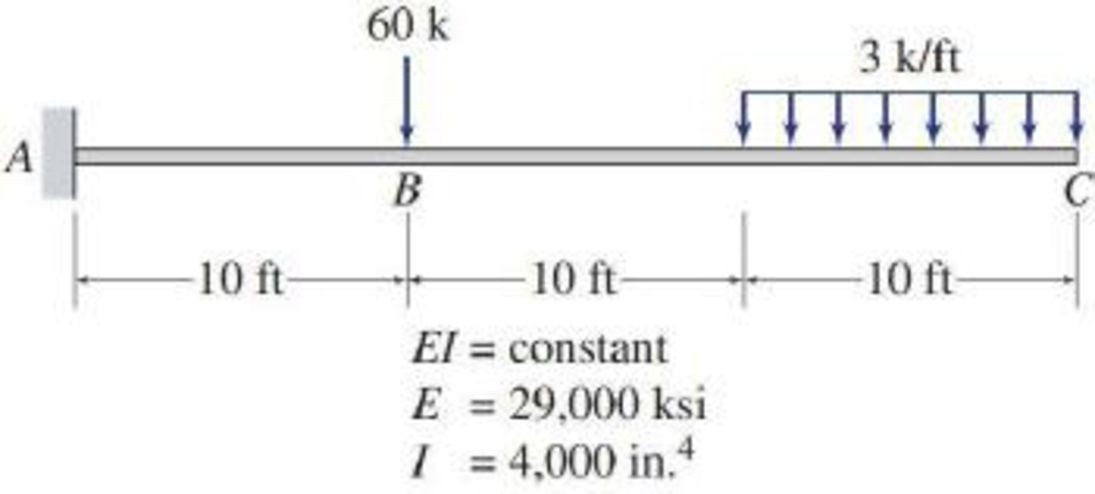# 6.40 through 6.43 Use the Conjugate-beam method to determine the slopes and deflections at points B and C of the beams Figs. P6.14 through P6.17. FIG. P6.16, P6.42

#### Solutions

Chapter
Section
Chapter 6, Problem 42P
Textbook Problem
264 views

## 6.40 through 6.43 Use the Conjugate-beam method to determine the slopes and deflections at points B and C of the beams Figs. P6.14 through P6.17.FIG. P6.16, P6.42To determine

Find the slope θB&θC and deflection ΔB&ΔC at point B and C of the given beam using the conjugate-beam method.

### Explanation of Solution

The Young’s modulus (E) is 29,000 ksi.

The moment of inertia (I) is 4,000in.4.

Calculation:

Consider elastic modulus E of the beam is constant.

Show free body diagram of the given beam as in Figure (1).

Refer Figure (1),

Consider upward is positive and downward is negative.

Consider clockwise is negative and counterclowise is positive.

Since support C is a free end there is no reaction.

V=0RA(60+(3×10))RA=90k

Determine the bending moment at A;

MA(3×10×102+20)(60×10)=0MA=1,350kipsft

Determine the bending moment at B;

MB1,350+(90×10)=0MB=1,350900MB=450kipsft

Determine the moment at D;

MD+90×20(60×10)1,350=0MB=1,800+1,950MB=150kipsft

Determine the bending moment at C;

MC1,350+(90×30)(60×20)(3×10×102)=0MC=2,700+2,700MC=0

Show the M/EI diagram for the given beam as in Figure (2).

Conjugate-beam method:

In the given beam system, point A is a fixed end and point C is free end. But in the conjugate-beam method the fixed end of a real beam becomes free and the free end of real beam changed into the fixed end.

Show the M/EI diagram for the conjugate-beam as in Figure (3).

Calculation of shear at B in the conjugate-beam:

The shear force at B of the conjugate beam is equal to the slope at B on the real beam.

Consider the external forces acting (left of B) upward on the free body diagram as positive.

Determine the shear force at B using the relation;

SB=(b1h1)(12×b2×h2)

Here, b is the width and h is the height of the triangle and rectangle.

Substitute 10 ft for b1, 450EI for h1, 10 ft for b2, and (1,350EI450EI) for h2.

SB=(10×450EI)(12×10×(1,350EI450EI))=9,000kips-ft2EI

Determine the deflection at point B using the relation;

θB=9,000kips-ft2EI

Substitute 29,000 ksi for E and 4,000in.4 for I.

Hence, the slope at point B is 0.0112rad_.

The bending moment at B in the conjugate beam is equal to defection at B on the real beam.

Take the clockwise moments of the external forces about B as positive.

Determine the bending moment B using the relation;

MB=(b1h1)(b2)(12×b2×h2)(23×b2)

Substitute 10 ft for b1, 450EI for h1, 10 ft for b2, and (1,350EI450EI) for h2.

MB=(10×450EI)(102)(12×10×(1,350EI450EI))(23×10)=52,500kips-ft3EI

Determine the deflection at point B using the formula;

ΔB=52,500kips-ft3EI

Substitute 29,000 ksi for E and 4,000in.4 for I.

ΔB=52,500kips-ft3×(12in.1ft)329,000×4,000=0

### Still sussing out bartleby?

Check out a sample textbook solution.

See a sample solution

#### The Solution to Your Study Problems

Bartleby provides explanations to thousands of textbook problems written by our experts, many with advanced degrees!

Get Started

Find more solutions based on key concepts
A family uses 80 gallons of hot water per day. The water is heated from a line temperature of 55 F to 140 F. Ca...

Engineering Fundamentals: An Introduction to Engineering (MindTap Course List)

List features that are common to most keyboards. Describe how to use a keyboard shortcut.

Enhanced Discovering Computers 2017 (Shelly Cashman Series) (MindTap Course List)

What is civil law, and what does it accomplish?

Principles of Information Security (MindTap Course List)

Describe the effect of pressure on an enclosed volume of a gas.

Automotive Technology: A Systems Approach (MindTap Course List)

If your motherboard supports ECC DDR3 memory, can you substitute non-ECC DDR3 memory?

A+ Guide to Hardware (Standalone Book) (MindTap Course List)

Describe an ideal tack weld.

Welding: Principles and Applications (MindTap Course List)